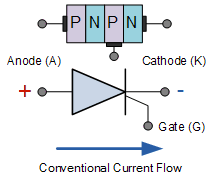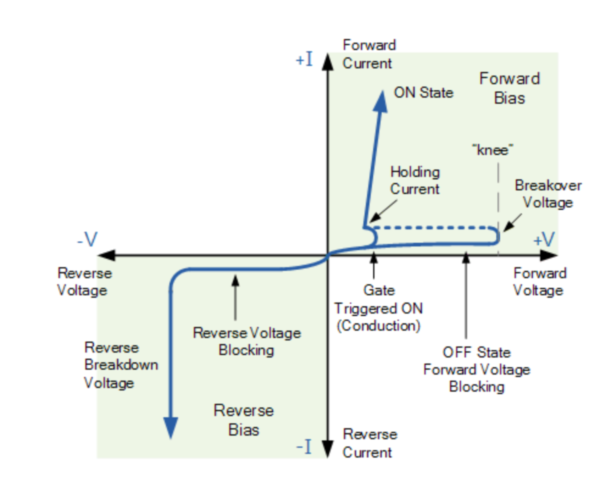# V-I Characteristics of SCR Explained In Detail

## V-I Characteristics of  SCR

In his article we will draw and explain the V-I characteristics of SCR in detail.

It is the curve between anode-cathode voltage (V) and anode current (I) of an SCR at constant gate current.

Fig.1 shows the V-I characteristics of a typical SCR .Fig.1

Please subscribe to Electronics Post Channel if you like my tutorials.

### Important Points About The V-I Characteristics of SCR

#### Forward Characteristics

When anode is positive w.r.t. cathode, the curve between V and I is called the forward characteristics.

In fig.1, OABC is the forward characteristics of SCR at IG=0.

If the supply voltage is increased from zero, a point reached (point A) when the SCR starts conducting.

Under this condition,the voltage across SCR suddenly drops as shown by dotted curve AB and most of supply voltage appears across the load resistance RL .

If proper gate current is made to flow, SCR can close at much smaller supply voltage.

#### Reverse Characteristics

When anode is negative w.r.t. cathode, the curve between V and I is known as reverse characteristics.

The reverse voltage does come across SCR when it is operated with a.c. supply.

If the reverse voltage is gradually increased, at first the anode current remains small (i.e. leakage current) and at some reverse voltage, avalanche breakdown occurs and the SCR starts conducting heavily in the reverse direction as shown by the curve DE.

This maximum reverse voltage at which SCR starts conducting heavily is known as reverse breakdown voltage.

### SCR in Normal Operation

In order to operate the SCR in normal operation, the following points are kept in view:

1. The supply voltage is generally much less than breakover voltage.
2. The SCR is turned on by passing appropriate amount of gate current ( a few mA) and not by breakover voltage.
3. When SCR is operated from a.c.  supply, the peak reverse voltage which comes during negative half-cycle should not exceed the reverse breakdown voltage.
4. When SCR is to be turned OFF from the ON state, anode current should be reduced to holding current.
5. If gate current is increased above the required value, the SCR will close at much reduced supply voltage.

### Important Terms In The V-I Characteristics of SCR

The following terms are much used in the study of SCR :

1. Breakover voltage
2. Peak reverse voltage
3. Holding current
4. Forward current rating
5. Circuit fusing rating

#### 1.  Breakover Voltage

It is the minimum forward voltage, gate being open, at which SCR starts conducting heavily i.e. turned on.

Thus, if the breakover voltage of an SCR is 200 V, it means that it can block a forward voltage  (i.e. SCR remains open) as long as the supply voltage is less than 200 V. If the supply voltage is more than this value, then SCR will be turned on.

In practice, the SCR is operated with supply voltage less than breakover voltage and it is then turned on by means of a small voltage applied to the gate.

Commercially available SCRs have breakover voltages from about 50 V to 500 V.

#### 2.  Peak Reverse Voltage (PRV)

It is the maximum reverse voltage  (cathode positive w.r.t. anode) that can be applied to an SCR without conducting in the reverse direction.

PRV is an important consideration while connecting an SCR in an a.c. circuit. During the negative half of a.c. supply, reverse voltage is applied across SCR. If PRV is exceeded, there may be avalanche breakdown and the SCR will be damaged if the external ciruit does not limit the current.

Commercially available SCRS have PRV ratings upto 2.5 kV.

#### 3.  Holding Current

It is the maximum anode current, gate being open, at which SCR is turned OFF from ON condition.

When SCR is in the conducting state, it can not be turned OFF even if gate voltage is removed.

The only way to turn off or open the SCR is to reduce the supply voltage to almost zero at which point the internal transistor comes out of saturation and opens the SCR.

The anode current under this condition is very small (a few mA) and is called holding current.

Thus, if an SCR has a holding current of 5mA, it means that if anode current is made less than 5 mA, then SCR will be turned off.

#### 4.  Forward Current Rating

It is the maximum anode current that an SCR is capable of passing without destruction.

Every SCR has a safe value of forward current which it can conduct. If the value of current exceeds this value, the SCR may be destroyed due to intensive heating at the junction.

For example, if an SCR has a forward current rating of 40 A, it means that the SCR can safely carry only 40 A. Any attempt to exceed this value will result in the destruction of the SCR.

Commercially available SCRs have forward current ratings from about 30A to 100A.

#### 5.  Circuit Fusing  (I2t) Rating

It is the product of square forward surge current and the time of duration of the surge i.e.,

Circuit fusing rating =I2t

The circuit fusing rating indicates the maximum forward surge current capability of SCR.

For example, consider an SCR having circuit fusing rating of 90 A2s. If this rating is exceeded in the SCR circuit , the device will be destroyed by excessive power dissipation.

You Might Like The Following Articles

Next Post : How To Test A Relay With A Multimeter